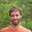Question-and-Answer Resource for the Building Energy Modeling Community
Get started with the Help page

Partially insulated wallHi,

I'm modeling a warehouse with some interior office spaces in eQUEST for LEED certification; normally not a complicated task. However with this model, I need to add insulation to the concrete tilt-up walls, but only at 10 feet AFF and above. Is there a way to specify the height at which the insulation should start? Or do I need to calculate some kind of averaged R-value for the whole wall?

Any suggestions would be a real help.

edit retag close merge delete

Sort by » oldest newest most votedYou may already be aware, but if you did add an averaged-R value for the whole wall, you wouldn't want to simply area-weight average the R-value. Rather, you'd want to area-weight average the U-value, then calculate the R-value from it, since heat transfer is proportional to U-value and not R-value.

For example, if you have 25% R-5 and 75% R-20, the answer is not: 0.25x5+0.75x20 = R-16.25

Rather, the answer is: 1/(0.25/5+0.75/10) = R-11.43

more

You can model this wall by creating two different walls at two different heights in the Detailed Edit mode of eQUEST. If you want to follow this path, I would recommend looking at the DOE2.2 Dictionary which is available on the eQUEST download page for details on how the geometry of exterior walls is defined. However, I think it would be much simpler to calculate the average R-value for the wall and stick with one wall type. I think that the differences in building loads and results would be very minor in either case.

more